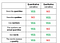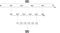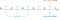# What Are Variables?

In statistics, a variable has two defining characteristics:

• A variable is an attribute that describes a person, place, thing, or idea.
• The value of the variable can “vary” from one entity to another.

Variables in statistics can describe either quantities, or qualities.

For instance, the `Height` variable for example describe tall of object. Generally, a variable that describes how much there is of something describes a quantity, and, for this reason, it’s called a quantitative variable. Usually, quantitative variables describe a quantity using real numbers, but there are also cases when words are used instead. Height, for example, can be described using real numbers, but it can also be described using labels like “tall” or “short”.

Variables that describe qualities are called qualitative variables or categorical variables. Generally, qualitative variables describe what or how something is.

Usually, qualitative variables describe qualities using words, but numbers can also be used. For instance, the number of a player’s shirt or the number of a racing car are described using numbers. The numbers don’t bear any quantitative meaning though, they are just names, not quantities.Comparison between Qualitative and Qualitative variables

The amount of information a variable provides depends on its nature (whether it’s quantitative or qualitative), and on the way it’s measured.

For instance, if we analyze the `Team` variable for any two individuals:

• We can tell whether or not the two individuals are different from each other with respect to the team they play.

But if there’s a difference:

• We can’t tell the size of the difference.
• We can’t tell the direction of the difference — we can’t say that team A is greater or less than team B.

On the other side, if we analyze the `Height` variable:

• We can tell whether or not two individuals are different.

If there’s a difference:

• We can tell the size of the difference. If player A has 190 cm and player B has 192 cm, then the difference between the two is 2 cm.
• We can tell the direction of the different from each perspective: player A has 2 cm less than player B, and player B has 2 cm more than player A.

The `Team` and `Height` variables provide different amounts of information because they have a different nature (one is qualitative, the other quantitative), and because they are measured differently. The system of rules that define how each variable is measured is called scale of measurement.

The characteristics of each scale pivot around three main questions:

• Can we tell whether two individuals are different?
• Can we tell the direction of the difference?
• Can we tell the size of the difference?

Variables on nominal scale

The `Team` variable is an example of a variable measured on a nominal scale. For any variable measured on a nominal scale these are its characteristics:

When a qualitative variable is described with numbers, the principles of the nominal scale still hold. We can tell whether there’s a difference or not between individuals, but we still can’t say anything about the size and the direction of the difference.

If basketball player A has the number 5 on her shirt, and player B has 8, we can tell they’re different with respect to shirt numbers, but it doesn’t make any sense to subtract the two values and quantify the difference as a 3. Nor it makes sense to say that B is greater than A. The numbers on the shirts are just identifiers here, they don’t quantify anything.

Variables on ordinal scale

variable shows labels like “short”, “medium”, or “tall”. By examining the values, we can tell whether two individuals are different or not. But, unlike in the case of a nominal scale, we can also tell the direction of the difference. Someone who is assigned the label “tall” has a bigger height than someone assigned the label “short”.

However, we still can’t determine the size of the difference. This is an example of a variable measured on an ordinal scale.

Generally, for any variable measured on an ordinal scale, we can tell whether individuals are different or not, we can also tell the direction of the difference, but we still can’t determine the size of the difference.

Common examples of variables measured on ordinal scales include ranks: ranks of athletes, of horses in a race, of people in various competitions

The values of the variables measured on an ordinal scale can be both words and numbers. When the values are numbers, they are usually ranks. But we still can’t use the numbers to compute the size of the difference. We can’t say how much faster an athlete was than another by simply comparing their ranks.

Whether a variable is quantitative or qualitative is independent of the way the variable is measured. The `Height` variable, for instance, is quantitative no matter how we measure it. The fact that we use words like "short" or "tall" doesn't change its underlying nature. The `Height` variable still describes a magnitude, but in a different way.

Variables on ratio scale

A variable measured on a scale that preserves the order between values and has well-defined intervals using real numbers is an example of a variable measured either on an interval scale, or on a ratio scale.

In practice, variables measured on interval or ratio scales are very common, if not the most common. Examples include:

• Height measured with a numerical unit of measurement (like inches or centimeters).
• Weight measured with a numerical unit of measurement.
• Time measured with a numerical unit of measurement.
• The price of various products measured with a numerical unit of measurement (like dollars, pounds, etc.).

On a ratio scale, the zero point means no quantity. For example, the `Weight` variable is measured on a ratio scale, which means that 0 grams indicate the absence of weight.

On an interval scale, however, the zero point doesn’t indicate the absence of a quantity. It actually indicates the presence of a quantity.

To exemplify this case using a data created for this purposed, we’ve used the `Weight` variable (measured on a ratio scale), and created a new variable that is measured on an interval scale. The new variable describes by how many kilograms the weight of a player is different than the average weight of the players in our data set. Here's a random sample that includes values from the new variable named `Weight_deviation`:

If a person had a value of 0 for `Weight_deviation` variable (which is measured on an interval scale), that wouldn't mean the player has no weight. Rather, it'd mean that her weight is exactly the same as the mean. The mean of the `Weight` variable is roughly 78.98 kg, which means that the zero point in the `Weight_deviation` variable is equivalent to 78.98 kg.

On the other side, a value of 0 for the `Weight` variable, which is measured on a ratio scale, indicates the absolute absence of weight.Another important difference between the two scales is given by the way we can measure the size of the differences.

On a ratio scale, we can quantify the difference in two ways. One way is to measure a distance between any two points by simply subtracting one from another. The other way is to measure the difference in terms of ratios.

For example, by doing a simple subtraction using the data in the table above, we can tell that the difference (the distance) in weight between Clarissa dos Santos and Alex Montgomery is 5 kg. In terms of ratios, however, Clarissa dos Santos is roughly 1.06 (the result of 89 kg divided by 84 kg) times heavier than Alex Montgomery. To give a straightforward example, if player A had 90 kg and player B had 45 kg, we could say that person A is two times (90 kg divided by 45 kg) heavier than person B.

On an interval scale, however, we can measure meaningfully the difference between any two points only by finding the distance between them (by subtracting one point from another). If we look at the weight deviation variable, we can say there’s a difference of 5 kg between Clarissa dos Santos and Alex Montgomery. However, if we took ratios, we’d have to say that Clarissa dos Santos is two times heavier than Alex Montgomery, which is not true.

In practice, variables measured on an interval scale are relatively rare.

# Definitions:

Discrete variables are countable in a finite amount of time. For example, you can count the change in your pocket. You can count the money in your bank account. You could also count the amount of money in everyone’s bank accounts. It might take you a long time to count that last item, but the point is it’s still countable.

Generally, if there’s no possible intermediate value between any two adjacent values of a variable, we call that variable discrete.

Continuous variable:are numeric variables that have an infinite number of values between any two values. A continuous variable can be numeric or date/time. For example, the length of a part or the date and time a payment is received.Continuous variable has infinite number of values

Generally, if there’s an infinity of values between any two values of a variable, we call that variable continuous.

Whether a variable is discrete or continuous is determined by the underlying nature of the variable being considered, and not by the values obtained from the measurement.

Generally, every value of a continuous variable is an interval, no matter how precise the value is. The boundaries of an interval are sometimes called real limits. The lower boundary of the interval is called lower real limit, and the upper boundary is called upper real limit. let’s dive deeper to clarify the concept of real limit.

Real limits of a continuous variable: Values that are above and below the recorded value by one-half of the smallest measuring unit of the scale

When data are comprised of interval/ratio numbers or class intervals, e.g., (20–29) (30–39) (40–49) and so on, the limits of such numbers or class intervals are understood in terms of “true (real) limits.” True/real limits are defined by the highest possible value — the upper limit — and the lowest possible value — the lower limit. The general rules for calculating the true limits of class intervals represented by numbers are:

Upper True Limit: Add a 5 to the decimal place to the right of the last number appearing in the highest value specified by the number in the class interval.

Lower True Limit: Subtract a 5 to the decimal place to the right of the last number appearing in the lowest value specified by the number in the class interval.

If the class intervals of a variable are defined by whole numbers, to find the upper limit we add .5 to the highest value specified by the category, and to find the lower limit we subtract .5 from the lowest value specified in the category. For example:Describing lower/upper and real limits

In the figure above we can see for example that 88.5 is halfway between 88 and 89. If we got a measurement of 88.5 kg in practice, but we want only integers in our data (hence zero decimals precision), you might wonder whether to assign the value to 88 or 89 kg. The answer is that 88.5 kg is exactly halfway between 88 and 89 kg, and it doesn’t necessarily belong to any of those two values. The assignment only depends on how you choose to round numbers: if you round up, then 88.5 kg will be assigned to 89 kg; if you round down, then the value will be assigned to 88 kg.

Discrete variable: There are no possible values between adjacent units on the scale.

In this article we divided variables into two big categories: quantitative and qualitative. We’ve seen that quantitative variables can be measured on ordinal, interval, or ratio scales.

Communications and Electronics Engineer graduate.quick-learning adaptable individual,strong analytical skills in order to drive successful business solutions.

## More from Mohamed Abdelrazek

Communications and Electronics Engineer graduate.quick-learning adaptable individual,strong analytical skills in order to drive successful business solutions.